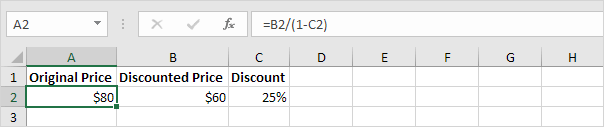# Discount

If you know the original price and the discounted price, you can calculate the percentage discount. If you know the original price and the percentage discount, you can calculate the discounted price, etc.

### Calculate Percentage Discount

If you know the original price and the discounted price, you can calculate the percentage discount.

1. First, divide the discounted price by the original price.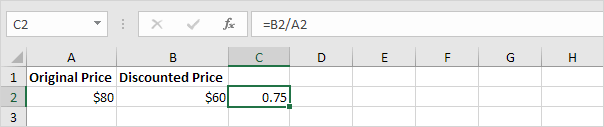Note: you're still paying \$60 of the original \$80. This equals 75%.

2. Subtract this result from 1.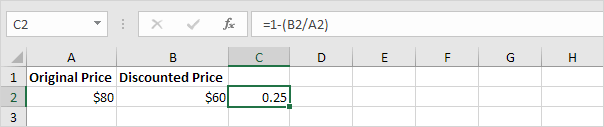Note: if you're still paying 75%, you're not paying 25% (the percentage discount).

3. On the Home tab, in the Number group, click the percentage symbol to apply a Percentage format.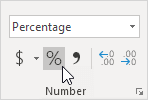Result.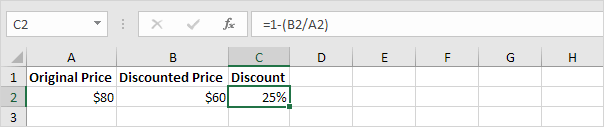### Calculate Discounted Price

If you know the original price and the percentage discount, you can calculate the discounted price.

1. First, subtract the percentage discount from 1.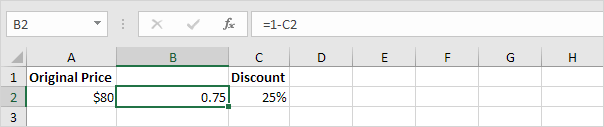Note: you're still paying 75%.

2. Multiply this result by the original price.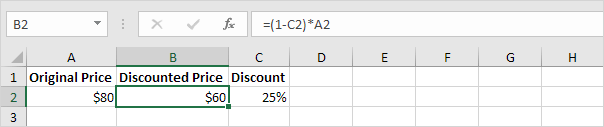Note: you're still paying 75% of the original \$80. This equals \$60.

### Calculate Original Price

If you know the discounted price and the percentage discount, you can calculate the original price. Take a look at the previous screenshot. To calculate the discounted price, we multiplied the original price by (1 - Percentage Discount).

1. To calculate the original price, simply divide the discounted price by (1 - Percentage Discount).